Test: Introduction to Heights & Distances

# Test: Introduction to Heights & Distances

Test Description

## 20 Questions MCQ Test Online MCQ Tests for Class 10 | Test: Introduction to Heights & Distances

Test: Introduction to Heights & Distances for Class 10 2023 is part of Online MCQ Tests for Class 10 preparation. The Test: Introduction to Heights & Distances questions and answers have been prepared according to the Class 10 exam syllabus.The Test: Introduction to Heights & Distances MCQs are made for Class 10 2023 Exam. Find important definitions, questions, notes, meanings, examples, exercises, MCQs and online tests for Test: Introduction to Heights & Distances below.
Solutions of Test: Introduction to Heights & Distances questions in English are available as part of our Online MCQ Tests for Class 10 for Class 10 & Test: Introduction to Heights & Distances solutions in Hindi for Online MCQ Tests for Class 10 course. Download more important topics, notes, lectures and mock test series for Class 10 Exam by signing up for free. Attempt Test: Introduction to Heights & Distances | 20 questions in 20 minutes | Mock test for Class 10 preparation | Free important questions MCQ to study Online MCQ Tests for Class 10 for Class 10 Exam | Download free PDF with solutions
 1 Crore+ students have signed up on EduRev. Have you?
Test: Introduction to Heights & Distances - Question 1

### When the sun's altitude changes from 30° to 60°, the length of the shadow of a tower decreases by 70m. What is the height of the tower?

Detailed Solution for Test: Introduction to Heights & Distances - Question 1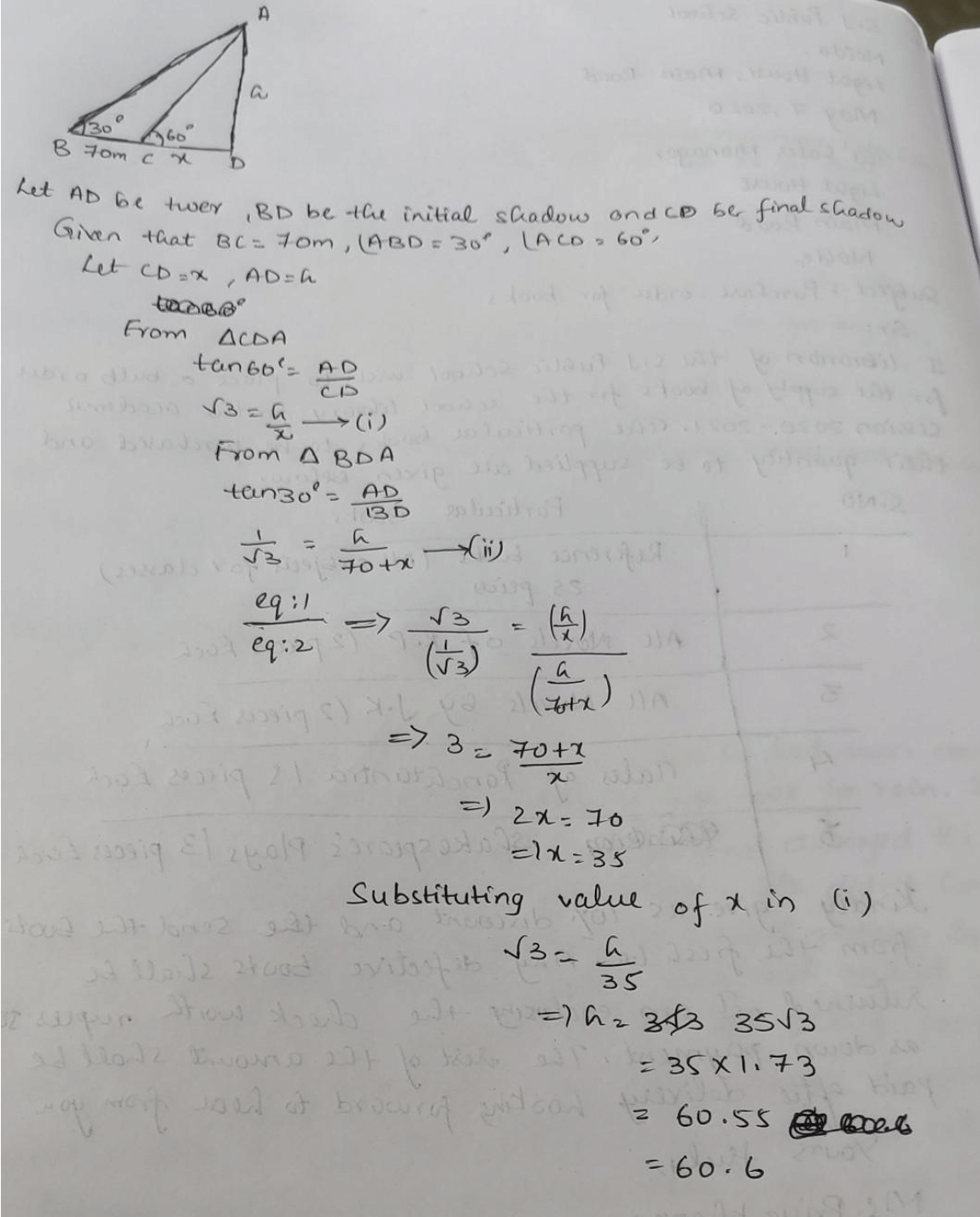Test: Introduction to Heights & Distances - Question 2

### The fig., shows the observation of point C from point A. The angle of depression from A is: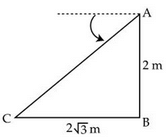​

Test: Introduction to Heights & Distances - Question 3

### From the given figure, find h.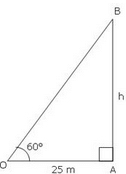​

Test: Introduction to Heights & Distances - Question 4

The angle formed by the line of sight with the horizontal, when the point being viewed is above the horizontal level is called:​

Detailed Solution for Test: Introduction to Heights & Distances - Question 4

The term angle of elevation denotes the angle from the horizontal upward to an object.  An observer’s line of sight would be above the horizontal. And The term angle of depression denotes the angle from the horizontal downward to an object.  An observer’s line of sight would be below the horizontal. So The angle is called Angle of elevation.

Test: Introduction to Heights & Distances - Question 5

A tower stands vertically on the ground from a point on the ground which is 25 m away from the foot of tower if the height of tower is 25√3 metres find the angle of elevation.​

Test: Introduction to Heights & Distances - Question 6

The angle of elevation of the sun, when the length of the shadow of a tree is equal to the height of the tree, is:

Detailed Solution for Test: Introduction to Heights & Distances - Question 6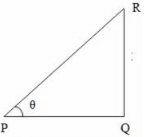Consider the diagram shown above where QR represents the tree and PQ represents its shadow

We have, QR = PQ
Let ∠QPR = θ
tan θ = QR/PQ = 1 (since QR = PQ)
⇒ θ = 45°

i,e., required angle of elevation = 45°

Test: Introduction to Heights & Distances - Question 7

If the length of a shadow cast by a pole is √3 times the length of the pole, then the angle of elevation of the sun is​

Detailed Solution for Test: Introduction to Heights & Distances - Question 7

Let AB be the tree and AC be its shadow.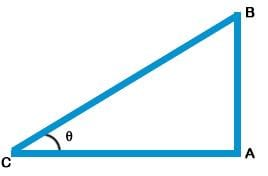Let ∠ACB = θ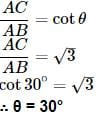Test: Introduction to Heights & Distances - Question 8

Let AC be the rope whose length is 20 m, and AB be the vertical pole of height h m and the angle of elevation of A at point C on the ground is 30°.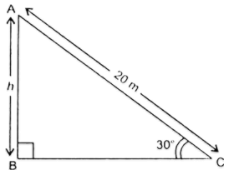Detailed Solution for Test: Introduction to Heights & Distances - Question 8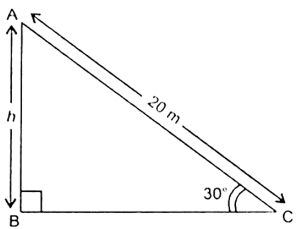Fif. 9.11
i.e., ∠ACB = 30°
In right triangle ABC, we have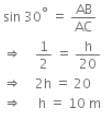Hence, the height of the pole is 10 m.

Test: Introduction to Heights & Distances - Question 9

A 20 m long ladder touches the wall at a height of 10 m. The angle which the ladder makes with the horizontal is

Test: Introduction to Heights & Distances - Question 10

The ratio of the length of a rod and its shadow is 1:1/√3. what is the angle of elevation of the source of light

Test: Introduction to Heights & Distances - Question 11

If sun’s elevation is 60° then a pole of height 6 m will cast a shadow of length

Test: Introduction to Heights & Distances - Question 12

The angle of elevation from a point 30 metre from the base of tree as level ground to the top of the tree is 60°. The height of the tree is :

Detailed Solution for Test: Introduction to Heights & Distances - Question 12

Angle of elevation is 60
Base = 30m
Height of the tree = Perpendicular
So in the right triangle
Where base is given and we have to find perpendicular we have only tan θ
So, Tan θ = P/B
Tan 60 = P/30
√30 = P/30
P = 30√30

Test: Introduction to Heights & Distances - Question 13

Tree is broken by the wind the top struck the ground at 30degree at a distance of 30m. away from the root. Find the height of the tree.

Detailed Solution for Test: Introduction to Heights & Distances - Question 13

Let BD be the whole height of the tree

The tree breaks at point C and makes ΔABC as given into the figure such that

AC = CD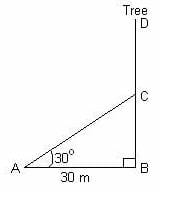In rt. ΔABC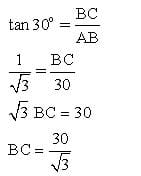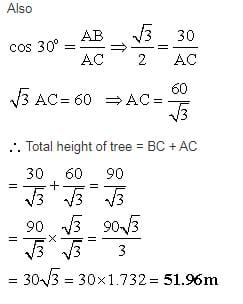Test: Introduction to Heights & Distances - Question 14

Find AB in the given figure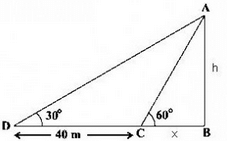Detailed Solution for Test: Introduction to Heights & Distances - Question 14

Use tanθ formula in both the triangles ABC and ABD.

Test: Introduction to Heights & Distances - Question 15

Guddi was standing on a road near a mall. She was 1000m away from the mall and able to see the top of the mall from the road in such a way that top of the tree, which is in between her and the mall, was exactly in line of sight with the top of the mall. The tree height is 10m and it is 20m away from Guddi. How tall is the mall?

Detailed Solution for Test: Introduction to Heights & Distances - Question 15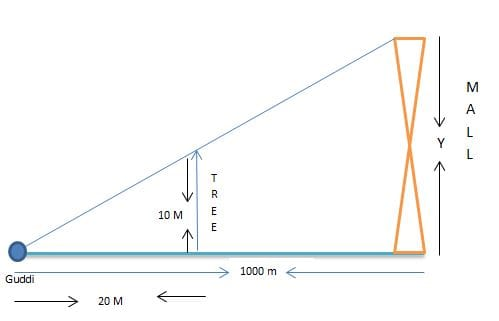Angle of elevation for Guddi is same for the tree and the mall.

So, the ratio of object height to its shadow will be same for the both subjects.

Therefore by proportionality rule,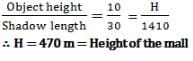Test: Introduction to Heights & Distances - Question 16

A tower stands vertically on the ground. From a point C on the ground, which is 20 m away from the foot of the tower, the angle of elevation of the top of the tower is found to be 450. The height of the tower is

Detailed Solution for Test: Introduction to Heights & Distances - Question 16

Angle of elevation =45 degrees
Base=20 m
Height of the tower=perpendicular
We have tan 45=P/B
1=P/20
P=20 m
Hence the height of the tower  is 20 metres.

Test: Introduction to Heights & Distances - Question 17

The angle of elevation from a point 30 feet from the base of a pole, of height h, as level ground to the top of the pole is 45o. Which equation can be used to find the height of the pole.

Test: Introduction to Heights & Distances - Question 18

A tree casts a shadow 4 m long on the ground, when the angle of elevation of the sun is 45°. The height of the tree is:​

Detailed Solution for Test: Introduction to Heights & Distances - Question 18

In a triangle with one angle being 90 degrees (which the tree makes with the ground) and the other being 45 degrees (the angle of elevation), the 3rd angle is bound to be 45 degrees (180 - 90 - 45 = 45).

We also know that sides opposite to equal angles are equal.

Hence, the height of the tree will also be 4m.

Test: Introduction to Heights & Distances - Question 19

The angle of elevation of the sun when the length of the shadow of the tree is √3 times the height of the tree is​

Test: Introduction to Heights & Distances - Question 20

A tower stands vertically on the ground. From a point on the ground which is 25 m away from the foot of the tower, the angle of elevation of the top of the tower is found to be 45o. Then the height (in meters) of the tower is​

Detailed Solution for Test: Introduction to Heights & Distances - Question 20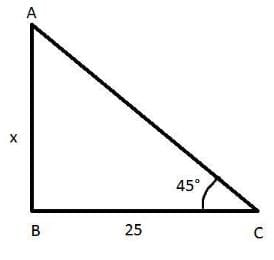A point on the ground which is 25 m away from the foot of the tower i. BC= 25 m

Let the height of the tower be x

The angle of elevation of the tower is found to be 45 degree.i.e.∠ACB=45°

In ΔABC

Using trigonometric ratios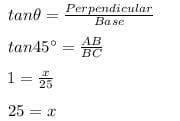Hence the height of the tower is 25 m.

## Online MCQ Tests for Class 10

615 tests
 Use Code STAYHOME200 and get INR 200 additional OFF Use Coupon Code
Information about Test: Introduction to Heights & Distances Page
In this test you can find the Exam questions for Test: Introduction to Heights & Distances solved & explained in the simplest way possible. Besides giving Questions and answers for Test: Introduction to Heights & Distances, EduRev gives you an ample number of Online tests for practice

615 tests